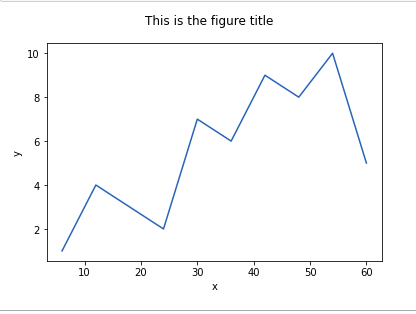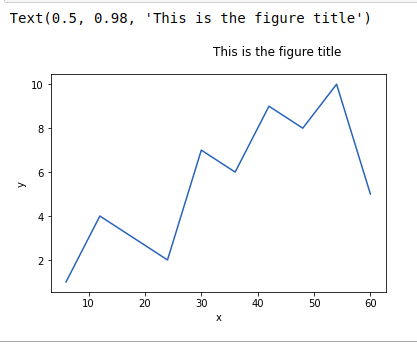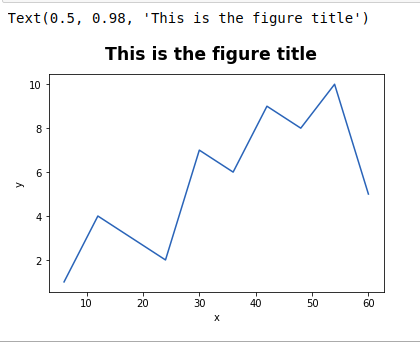Related Articles
Matplotlib.pyplot.suptitle() function in Python
• Last Updated : 28 Jul, 2020

Matplotlib is a library in Python and it is a mathematical extension for NumPy library. Pyplot is a state-based interface to a Matplotlib module which provides a MATLAB-like interface.

## matplotlib.pyplot.suptitle() Function

The suptitle() function in pyplot module of the matplotlib library is used to add a title to the figure.

Syntax: matplotlib.pyplot.suptitle(t, **kwargs)

Parameters: This function will have following parameters:

• t : The title text you want to add to your graph.
• x : The x location of the text in figure coordinates. It’s default value is 0.5.
• y : The y location of the text in figure coordinates. It’s default value is 0.98.
• horizontalalignment (ha)  : {‘center’, ‘left’, right’}, The horizontal alignment of the text is relative to (x, y). It’s default value is ‘center’.
• verticalalignment (va) : {‘top’, ‘center’, ‘bottom’, ‘baseline’}, The vertical alignment of the text is relative to (x, y). It’s default value is ‘top’.
• fontsize, size : {size in points, ‘xx-small’, ‘x-small’, ‘small’, ‘medium’, ‘large’, ‘x-large’, ‘xx-large’}, The font size of the text. It’s default value is ‘large’.
• fontweight, weight : {a numeric value in range 0-1000, ‘ultralight’, ‘light’, ‘normal’, ‘regular’, ‘book’, ‘medium’, ‘roman’, ‘semibold’, ‘demibold’, ‘demi’, ‘bold’, ‘heavy’, ‘extra bold’, ‘black’}, The font weight of the text. It’s default value is ‘normal’.
• fontproperties : None or dict, A dict of font properties. If fontproperties is given the default values for font size and weight are taken from the FontProperties defaults. rcParams[“figure.titlesize”] = ‘large’ and rcParams[“figure.titleweight”] = ‘normal’ are ignored in this case.
• **kwargs : Additional kwargs are matplotlib.text.Text properties.

Returns: The Text instance of the title.

Below examples illustrate the matplotlib.pyplot.suptitle() function in matplotlib.pyplot:

Example 1: Adding a title to the graph with font size 12.

## Python3

 `# importing matplotlib.pyplot module ` `import` `matplotlib.pyplot as plt ` ` `  `# values of x and y axes ` `x ``=` `[``6``, ``12``, ``18``, ` `     ``24``, ``30``, ``36``, ` `     ``42``, ``48``, ``54``, ` `     ``60``] ` `y ``=` `[``1``, ``4``, ``3``, ` `     ``2``, ``7``, ``6``, ` `     ``9``, ``8``, ``10``, ` `     ``5``] ` ` `  `# plotting the graph  ` `plt.plot(x, y) ` ` `  `# labelling axes ` `plt.xlabel(``'x'``) ` `plt.ylabel(``'y'``) ` ` `  `# adding title to the graph ` `# with font size 12 ` `plt.suptitle(``'This is the figure title'``,  ` `             ``fontsize ``=` `12``) ` ` `  `# show the plot ` `plt.show()`

Output :Example 2: Adding title to the graph with left horizontal alignment and font size 12.

## Python3

 `# importing matplotlib.pyplot module ` `import` `matplotlib.pyplot as plt ` ` `  `# values of x and y axes ` `x ``=` `[``6``, ``12``, ``18``, ` `     ``24``, ``30``, ``36``, ` `     ``42``, ``48``, ``54``, ` `     ``60``] ` ` `  `y ``=` `[``1``, ``4``, ``3``, ` `     ``2``, ``7``, ``6``, ` `     ``9``, ``8``, ``10``, ` `     ``5``] ` ` `  `# plotting the graph  ` `plt.plot(x, y) ` ` `  `# labelling axes ` `plt.xlabel(``'x'``) ` `plt.ylabel(``'y'``) ` ` `  `# Adding title to the graph  ` `# with left horizontal alignment ` `# and font size 12. ` `plt.suptitle(``'This is the figure title'``,  ` `             ``ha ``=` `'left'``, ` `             ``fontsize ``=` `12``)`

Output:Example 3: Adding title to the graph with extra bold font weight and large font size.

## Python3

 `# importing matplotlib.pyplot module ` `import` `matplotlib.pyplot as plt ` ` `  `# values of x and y axes ` `x ``=` `[``6``, ``12``, ``18``, ` `     ``24``, ``30``, ``36``, ` `     ``42``, ``48``, ``54``, ` `     ``60``] ` ` `  `y ``=` `[``1``, ``4``, ``3``, ` `     ``2``, ``7``, ``6``, ` `     ``9``, ``8``, ``10``, ` `     ``5``] ` ` `  `# plotting the graph  ` `plt.plot(x, y) ` ` `  `# labelling axes ` `plt.xlabel(``'x'``) ` `plt.ylabel(``'y'``) ` ` `  `# Adding title to the graph  ` `# with extra bold font weight ` `# and large font size. ` `plt.suptitle(``'This is the figure title'``,  ` `             ``fontsize ``=` `'xx-large'``, ` `             ``weight ``=` `'extra bold'``)`

Output:Attention geek! Strengthen your foundations with the Python Programming Foundation Course and learn the basics.

To begin with, your interview preparations Enhance your Data Structures concepts with the Python DS Course.

My Personal Notes arrow_drop_up
Recommended Articles
Page :